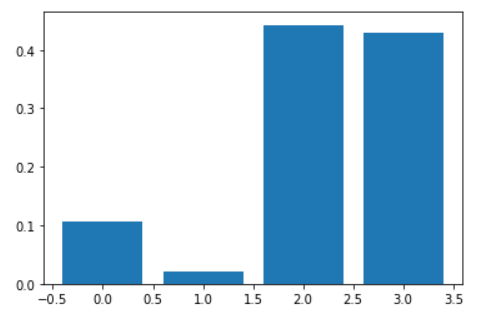Search
•Ekta Aggarwal

# Random Forests in Python

In this tutorial we would be understanding how to implement Random Forests algorithm in Python.

If you wish to understand the theory behind Random forests then you can refer to this tutorial: Random Forests Explained.

To run Random Forests in Python we will use iris dataset, which is inbuilt in Python. Iris dataset comprises of data for 150 flowers belonging to 3 different species: Setosa, Versicolor and Virginica. For these 150 flowers their Sepal Length, Sepal Width, Petal Length and Petal Width information is available.

Let us firstly load pandas library

`import pandas as pd`

Now we will load iris dataset from sklearn library

```from sklearn.datasets import load_iris

Following are the variable names in iris dataset

`iris.feature_names`

Output: ['sepal length (cm)', 'sepal width (cm)', 'petal length (cm)', 'petal width (cm)']

Now we are storing the independent variables from iris dataset in X and dependent variable in y

```X = iris.data
y = iris.target```

We can see from the shape that X has 150 rows and 4 columns

`X.shape`

Output:

(150, 4)

We can see the number of occurences of different species:

`pd.Series(y).value_counts()`

Output:

2 50 1 50 0 50 dtype: int64

Now we are splitting the data in training set and test set. Note that we will build our model using the training set and we will use test set to check our performance of the algorithm. We are splitting our data into 80% training set and 20% test set. We can see that training set has got 120 rows and test set has 30 rows.

```from sklearn.model_selection import train_test_split
X_train, X_test, y_train, y_test = train_test_split(X, y, test_size=0.2, random_state=42)

print(X_train.shape);

print(X_test.shape);

print(y_train.shape);

print(y_test.shape)

```

Output:

(120, 4) (30, 4) (120,) (30,)

### Building Random Forests with default parameters

Let us build our Random Forest model with default parameters. With the following code we are loading our RandomForestClassifier function from ensemble module in Python. For regression problem we would have used RandomForestRegressor.

`from sklearn.ensemble import RandomForestClassifier`

Defining our Random Forests model with default parameters and fitting it on training set.

```rf = RandomForestClassifier()
rf.fit(X_train, y_train)```

Making the predictions on test set:

`pred_test = rf.predict(X_test)`

We are not getting the accuracy for our test sets.

```from sklearn.metrics import accuracy_score
accuracy_score(y_test, pred_test)```

Output: 1.0

### Optimising the hyper parameters

The most optimal way to find optimal parameters for our Random Forests model is by using GridSearch.

Here we are defining the dictionary for various parameters which we want to tune.

In Python following 5 parameters can be used for hypertuning Random Forests:

• n_estimators: Number of trees in the forest.

• max_depth: The maximum depth of the tree.

• min_samples_split: The minimum number of samples required to split a node

• min_samples_leaf: The minimum number of samples required to be at a leaf node. Let us say min_sample_leaf = 5, thus, If after splitting a node we do not have 5 observations in the child nodes then the parent node will not split.

• max_features: The number of features to consider while building a decision tree in the forest.

Currently we are only tuning n_estimators and max_depth:

```param_grid = {'n_estimators': [50,100,250,500],  'max_depth': [4, 8,10]}
print(param_grid)```

Now we are defining the model to use. random_state = 5 is used to create the model for reproducibility.

`model_RF = RandomForestClassifier(random_state=5)`

We are now defining the Grid Search for our model, specifying our parameter grid. CV = 10 implies 10-fold cross validation with scoring mechanism as 'accuracy'.

`model2 = GridSearchCV(estimator=model_RF, param_grid=param_grid, cv=10, scoring='accuracy', n_jobs=-1)`

We are not fitting the model to our training data to obtain the best parameters

`model2.fit(X_train, y_train)`

The best parameters of our model are given as:

`model2.best_params_`

Output: {'max_depth': 4, 'n_estimators': 250}

The best 10-fold cross validation accuracy for our model is:

`model2.best_score_`

Output: 0.9333333333333332

`model2.best_estimator_`

Output: RandomForestClassifier(max_depth=4, n_estimators=250, random_state=5)

Now we are fitting out final Random Forests model using our best estimator i.e.,

```rf = model2.best_estimator_
rf.fit(X_train,y_train)```

We are not getting the confusion matrix and accuracy for our training and test sets.

`from sklearn.metrics import confusion_matrix,accuracy_score`

### Making the predictions on training set:

`y_pred_RF_train = rf.predict(X_train)`

Creating the confusion matrix for training set:

```RF_train_ct = pd.DataFrame(confusion_matrix(y_train, y_pred_RF_train))
RF_train_ct```Now we are getting the accuracy for training set:

`accuracy_score(y_train, y_pred_RF_train)`

Output: 0.975

### Making the predictions on test set:

`y_pred_RF = rf.predict(X_test)`

Creating the confusion matrix for testset:

```RF_test_ct = pd.DataFrame(confusion_matrix(y_test, y_pred_RF))
RF_test_ct```Now we are getting the accuracy for test set:

`accuracy_score(y_test, y_pred_RF)`

Output: 1.0

### Variable Importance

Random Forests come with an inbuilt function feature_importances_ which provides the importance of the feature. The higher the feature importance, the more important is the variable.

Following code prints the feature importance of all the variables. We can see that features 3 and 4 are most important variables for our model.

```importance = rf.feature_importances_

for i,v in enumerate(importance):
print('Feature: %0d, Score: %.5f' % (i,v))```

Output: Feature: 0, Score: 0.10596 Feature: 1, Score: 0.02183 Feature: 2, Score: 0.44278 Feature: 3, Score: 0.42943

The code below plots the feature importance of various variables:

```import matplotlib.pyplot as plt

plt.bar([x for x in range(len(importance))], importance)
plt.show()```Tags:

See All